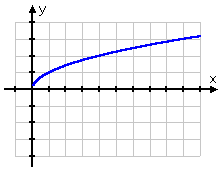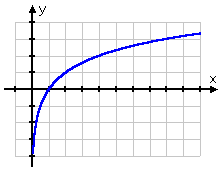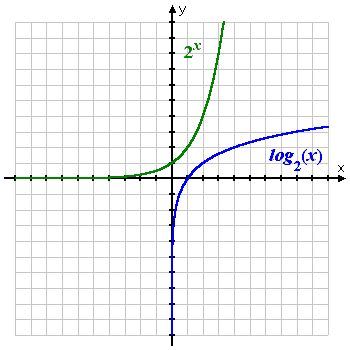Search

The Common and Natural Logarithms (page 3 of 3)

Sections: Introduction to logs, Simplifying log expressions, Common and natural logsA logarithm can have any positive value as its base, but two log bases are more useful than the others. The base-10, or "common", log is popular for historical reasons, and is usually written as "log(x)". For instance, pH (the measure of a substance's acidity or alkalinity), decibels (the measure of sound intensity), and the Richter scale (the measure of earthquake intensity) all involve base-10 logs. If a log has no base written, you should generally (in algebra classes) assume that the base is 10.

The other important log is the "natural", or base-e, log, denoted as "ln(x)" and usually pronounced as "ell-enn-of-x". (Note: That's "ell-enn", not "one-enn" or "eye-enn"!) Just as the number e arises naturally in math and the sciences, so also does the natural log, which is why you need to be familiar with it.

Warning: If you eventually progress to much-more advanced mathematics, you may find that sometimes "log(x)" means the base-e log or even base-2 log, rather than the common log.

Because the common and natural logs are pretty much the only logs that are used "in real life", these are the only two for which you have calculator keys. Make sure you know where these keys are, and how to use them.

Aside: Why would the "natural log" be denoted by "ln", rather than by "nl"? One popular idea relates to Euler ("OY-lur"), the guy who discovered (invented?) the natural exponential. Euler was Swiss and spoke French, so he might have called the function "le Logarithme Naturel", rather than "the natural log", in which case, "ln" makes sense. However, history shows that Euler actually used just "l(x)" for the logarithm using "his" number e as its base.

The first published use of the "ln" notation for the base-e logarithm was Stringham's, in his 1893 text "Uniplanar Algebra". Prof. Stringham was an American, so I have no idea why he would have used the notation "ln", other than perhaps to reflect a common, though mistaken, idea that Napier's log was a base-e log. That is, "ln" might have meant to stand for "Log of Napier".

It is even possible that the "ln" notation is a mistake: A European mathematician once proposed abbreviating "log" as "lg" and then using a script "l" for the function, with, effectively, a little squiggle afterwards which was meant to indicate a subscripted italic "g". But in the graphic I've seen, the "g" looks much more like an "n". Some of the other proposed notations for the natural logarithm were even more odd.

Mercator (not the map guy) used a Latin form of the term, "log naturalis" in his 1668 book on logarithms, and, as of the late 1800s, various English-speakers were using the notation "log.nat." for the natural logarithm. In short, the origin of this notation seems somewhat obscure, perhaps even unknown, which is unusual for such a recent "invention".

• Simplify log(100).

Since 100 = 102, then log(100) = log(102) = 2, because "log(100) = y" means "10 y = 100 = 102", so y = 2.

log(100) = 2

• Simplify log(98).

Since 98 is not a nice neat power of 10 (the way that 100 was), I cannot be clever with exponents to arrive at an exact answer. On this exercise, I am stuck with using my calculator to get an approximate value. So I'll plug this into my calculator, remembering to use the "LOG" key (not the "LN" key), and I get log(98) = 1.99122607569..., or:

log(98) = 1.99, rounded to two decimal places

• Simplify ln(e4.5).

Remember that "ln( )" means the base-e log, so "ln(e4.5)" might be thought of as "loge(e4.5)". The Relationship says that "ln(e4.5) = y" means "e y = e4.5", so y = 4.5, and:

ln(e4.5) = 4.5

Plug "ln(e4.5)" into your calculator, and you'll get the same answer. (Make sure you put parentheses around the "e4.5", so the calculator knows that the exponent is inside the log.)

Since 2 is a nice neat whole number and since e isn't, then it is unlikely that 2 is a nice neat power of e. So I can't simplify this expression by being clever with exponents. Instead, I'll have to evaluate this in my calculator, getting an approximate answer of ln(2) = 0.69314718056..., or:

ln(2) = 0.69, rounded to two decimal places.

The graph of a logarithm looks similar to that of a square root:

 square-root function log functionHowever, the square-root graph stops at the point (0, 0), while the logarithm graph does not pass through the origin, but instead passes through (1, 0) and then continues down along the right-hand (positive) side of the y-axis. Since the log function is the inverse of the exponential function, the graph of the log is the flip of the graph of the exponential:The exponential rides along the top of the x-axis, crosses the y-axis at the point (0, 1), and then shoots up. The logarithm rides up the right side of the y-axis, crosses the x-axis at the point (1, 0), and then shoots right. For more information, review the graphing lesson.

<< Previous  Top  |  1 | 2 | 3  |  Return to Index

 Cite this article as: Stapel, Elizabeth. "The Common and Natural Logarithms." Purplemath. Available from     https://www.purplemath.com/modules/logs3.htm. Accessed [Date] [Month] 2016

Study Skills Survey

Tutoring from Purplemath
Find a local math tutor